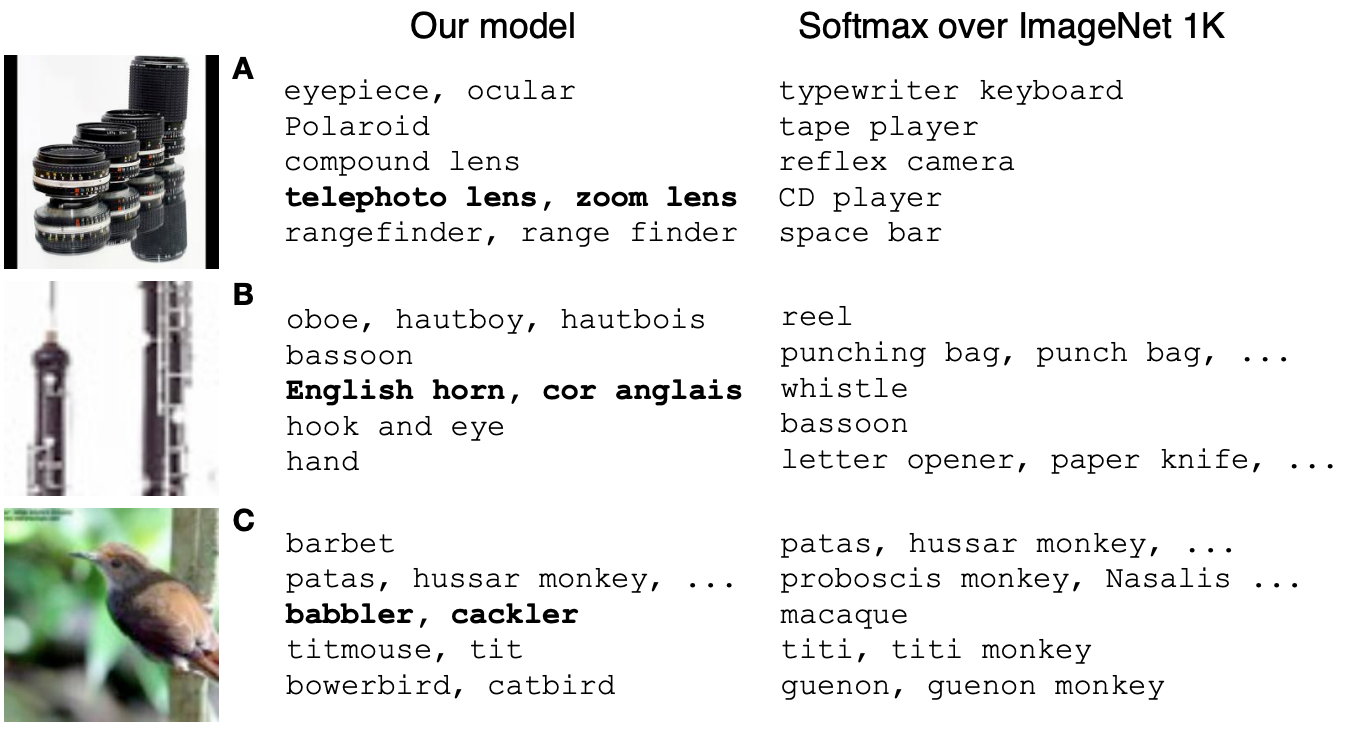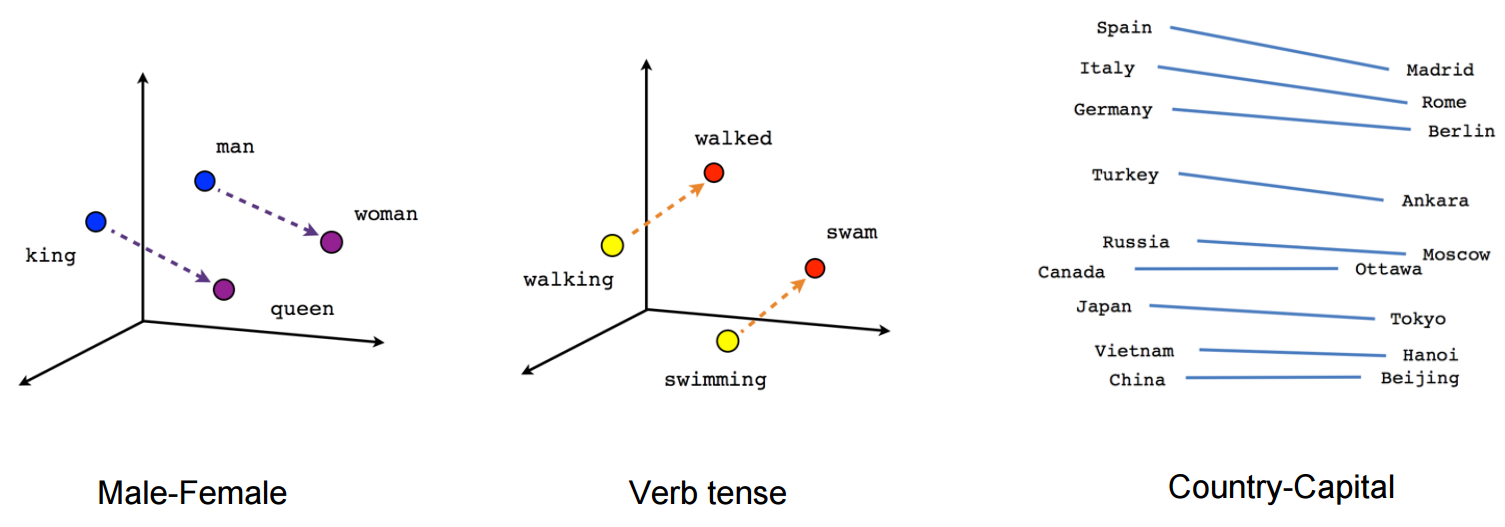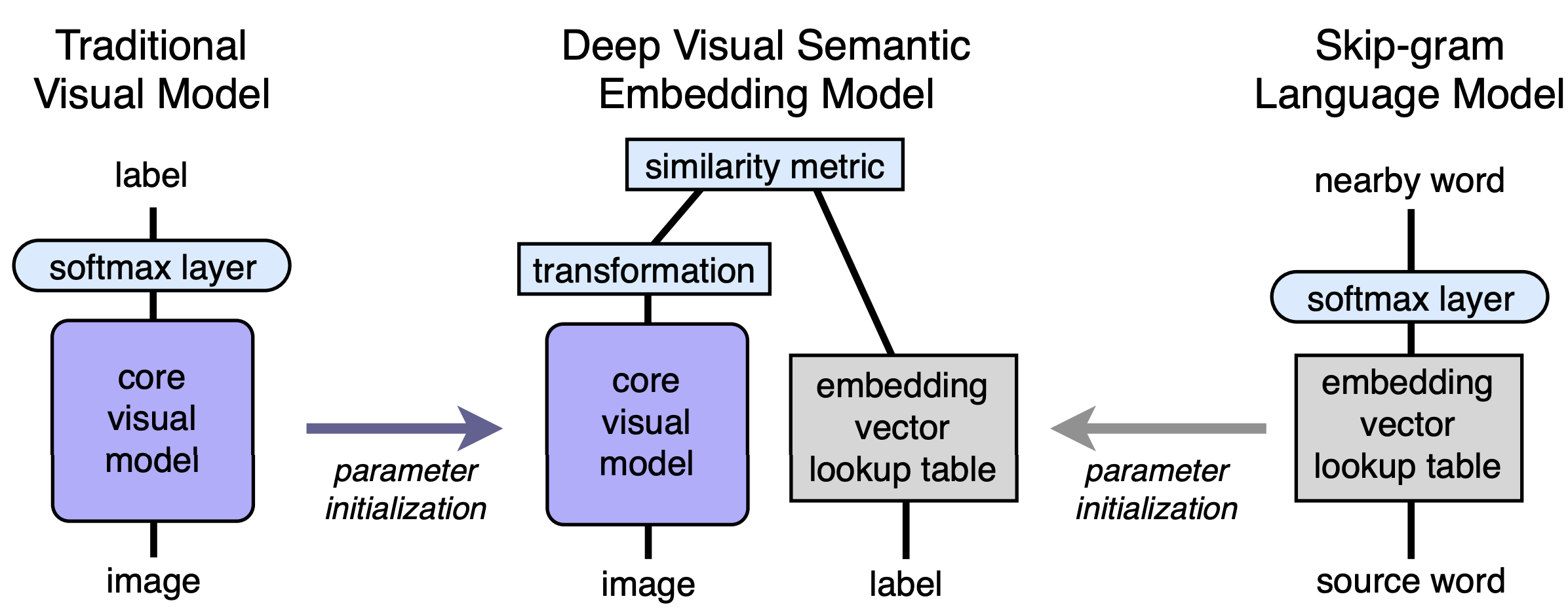# Deep Visual-Semantic Embedding Model with Keras

The image classification problem focus on classifying an image using a fixed set of labels. So they obviously do not scale and Furthermode, if a provided image has nothing to do with the original training set, the classifier will still attribute one or many of those labels to it. E.g. classifying a chicken image as digit five like in this model.

The Deep Visual-Semantic Embedding Model or DeViSE, mixes words and images to identify objects using both labeled image data as well as semantic information. Thus creating completely new ways of classifying images that can scale to larger number of labels which are not available during training. It does so by embedding the labels from ImageNet into a Word2Vec, thus levaraging the textual data to learn semantic relationships between labels, and explicitly maps images into a rich semantic embedding space.

In the remaining we will build DeViSE model in Keras### Data

Modern visual recognition systems are often limited in their ability to scale to large numbers of object categories.

This limitation is in part due to the increasing difficulty of acquiring sufficient training data in the form of labeled images as the number of object categories grows

One remedy is to leverage data from other sources – such as text data – both to train visual models and to constrain their predictions. In this paper we present a new deep visual-semantic embedding model trained to identify visual objects using both labeled image data as well as semantic information gleaned from unannotated text. W

The goal of the DeViSE is to leverage semantic knowledge learned in the text domain, and transfer it to a model trained for visual object recognition.

We begin by pre-training a simple neural language model wellsuited for learning semantically-meaningful, dense vector representations of words . In parallel, we pre-train a state-of-the-art deep neural network for visual object recognition , complete with a traditional softmax output layer. We then construct a deep visual-semantic model by taking the lower layers of the pre-trained visual object recognition network and re-training them to predict the vector representation of the image label text as learned by the language model. These three training phases are detailed below.### Architecture

The DeViSE model (as depicted in the following picture) is trained in three phases. A skip-gram word2vec model trained on wikipedia for instance. Separately a softmax ImageNet classifier and finally the two are combined into the DeViSE model.The object recognition neural network is pre-trained so that instead of predicting an image categiry, it will predict a vector representation of this category that match the represenatations predicted by the language model. In our case this translated into the following implementation:

• For the left model in the picture above, we use a pre-trained imagenet classifer (as described here).
• For the right model in the picture above, we use a pre-trained wordnet embedding layer for English from Facebook’s FastText.
• For the combination, we will replace the few last layers (i.e. the layers responsible of generating features for classification into 1K label) in the imagenet classifier by a new head that output a linear result matching the size of the word vector representation in the FastText word2vec for english.

In Keras, this architected is implemented as follows:

### Loss function

The loss function in the DeViSE paper, is defined as follows:

$loss(image, label) = \sum_{j \neq label} max[0, margin − \vec{t}_{label} M \vec{v} (image) + \vec{t}_{j} M \vec{v} (image)]$
• $$\vec{v}(image)$$ denotes the output column vector, for the given image, of the core visual network
• $$M$$ is the matrix of trainable parameters in the linear transformation layer
• $$\vec{t}_{label}$$ denotes the learned row embedding vector for the provided text label
• $$\vec{t}_{j}$$ denotes the embeddings of other text terms

For simplification we use the following formulas $$D_{C}(A,B)=1-S_{C}(A,B)$$ where $$D_{C}$$ is the Cosine Distance and $$S_{C}$$ is the Cosine Similarity. While $$A$$ and $$B$$ denotes the embedding vectors for the original and predcited labels (i.e. $$\vec{v}(image)$$). In Tensorflow, this loss function is implemented as:

### prediction

For evaluation the plain visual model + softmax was used as a baseline. Additionally a DeViSE model with randomized word embeddings was trained as a point of comparison, to validate that any benefits in the full model were in fact coming from information in the embedding layer (this was indeed the case). DeViSE works as intended, returning more sensible guesses compared to baseline, when considered qualitatively (see figure above). Interestingly DeViSE did a little bit worse than baseline on flat “hit@k” metrics (the probability of returning the true label in the top k predictions). To see the qualitative benefits empirically, the authors used a hierarchical “precision@k” metric that accepted results from an expanded list of valid labels derived from ImageNet’s label hierarchy. On this metric DeViSE did up to 7% better than baseline.

Full notebook can be found here - link